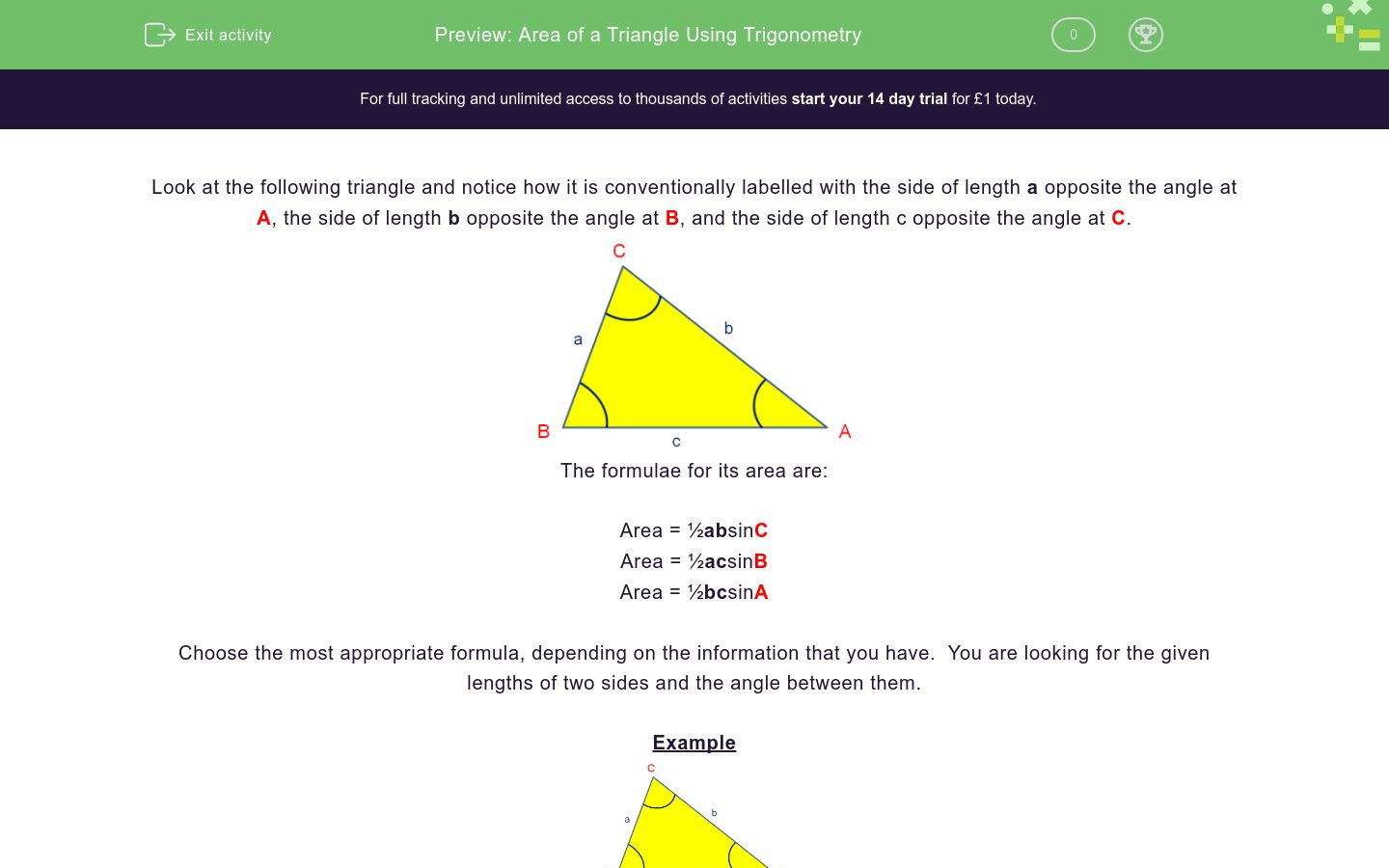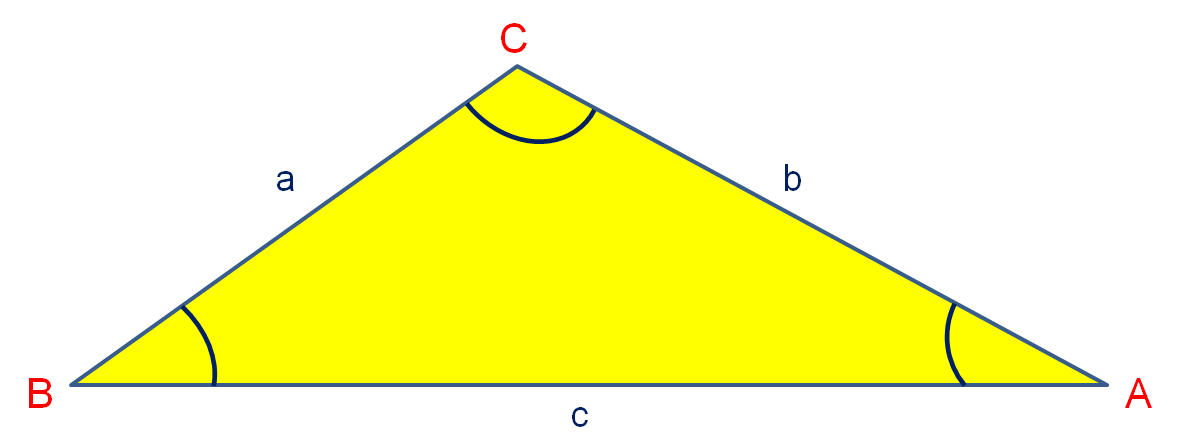# Area of a Triangle Using Trigonometry

In this worksheet, students use the trigonometrical formula to calculate the area of a triangle.Key stage:  KS 4

Curriculum topic:  Geometry and Measures

Difficulty level:### QUESTION 1 of 10

Look at the following triangle and notice how it is conventionally labelled with the side of length a opposite the angle at A, the side of length b opposite the angle at B, and the side of length c opposite the angle at C.The formulae for its area are:

Area = ½absinC

Area = ½acsinB

Area = ½bcsinA

Choose the most appropriate formula, depending on the information that you have.  You are looking for the given lengths of two sides and the angle between them.

ExampleIn the triangle above,

b = 4.3 cm

c = 5.1 cm

A = 38º

Use a trigonometrical formula, to calculate its area to 3 s.f.

Using the given information, we can only use the formula Area = ½ bcsinA.

Area = ½ x 4.3 x 5.1 x sin38º

Area = 0.5 x 4.3 x 5.1 x 0.616 = 6.7507... ≈ 6.75 cm2 (3 s.f.)In △ABC above,

b = 4 cm

c = 5 cm

A = 40º

Use a trigonometrical formula, to calculate its area in cm2 to 3 s.f.

(Just write the number)In △ABC above,

a = 4 cm

b = 6 cm

C = 55º

Use a trigonometrical formula, to calculate its area in cm2 to 3 s.f.

(Just write the number)In △ABC above,

a = 4.5 cm

c = 6 cm

B = 85º

Use a trigonometrical formula, to calculate its area in cm2 to 3 s.f.

(Just write the number)In △ABC above,

a = 6.2 cm

b = 7 cm

C = 125º

Use a trigonometrical formula, to calculate its area in cm2 to 3 s.f.

(Just write the number)In △ABC above,

a = 35 cm

b = 59 cm

C = 61º

Use a trigonometrical formula, to calculate its area in cm2 to 3 s.f.

(Just write the number)In △ABC above,

c = 22 cm

b = 35 cm

A = 40º

Use a trigonometrical formula, to calculate its area in cm2 to 3 s.f.

(Just write the number)In △ABC above,

c = 14 cm

a = 9.5 cm

B = 80.5º

Use a trigonometrical formula, to calculate its area in cm2 to 3 s.f.

(Just write the number)In △ABC above,

a = 12 cm

b = 11.5 cm

C = 115º

Use a trigonometrical formula, to calculate its area in cm2 to 3 s.f.

(Just write the number)In △ABC above,

a = 7.5 cm

b = 5.2 cm

C = 65º

Use a trigonometrical formula, to calculate its area in cm2 to 3 s.f.

(Just write the number)In △ABC above,

a = 31 mm

b = 74 mm

C = 122º

Use a trigonometrical formula, to calculate its area in cm2 to 3 s.f.

(Just write the number)

• Question 1In △ABC above,

b = 4 cm

c = 5 cm

A = 40º

Use a trigonometrical formula, to calculate its area in cm2 to 3 s.f.

(Just write the number)

6.43
EDDIE SAYS
Area = ½ × 4 × 5 × sin40°
• Question 2In △ABC above,

a = 4 cm

b = 6 cm

C = 55º

Use a trigonometrical formula, to calculate its area in cm2 to 3 s.f.

(Just write the number)

9.83
EDDIE SAYS
Area = ½ × 4 × 6 × sin55°
• Question 3In △ABC above,

a = 4.5 cm

c = 6 cm

B = 85º

Use a trigonometrical formula, to calculate its area in cm2 to 3 s.f.

(Just write the number)

13.4
EDDIE SAYS
Area = ½ × 4.5 × 6 × sin85°
• Question 4In △ABC above,

a = 6.2 cm

b = 7 cm

C = 125º

Use a trigonometrical formula, to calculate its area in cm2 to 3 s.f.

(Just write the number)

17.8
EDDIE SAYS
Area = ½ × 6.2 × 7 × sin125°
• Question 5In △ABC above,

a = 35 cm

b = 59 cm

C = 61º

Use a trigonometrical formula, to calculate its area in cm2 to 3 s.f.

(Just write the number)

903
EDDIE SAYS
Area = ½ × 35 × 59 × sin61°
• Question 6In △ABC above,

c = 22 cm

b = 35 cm

A = 40º

Use a trigonometrical formula, to calculate its area in cm2 to 3 s.f.

(Just write the number)

247
EDDIE SAYS
Area = ½ × 35 × 22 × sin40°
• Question 7In △ABC above,

c = 14 cm

a = 9.5 cm

B = 80.5º

Use a trigonometrical formula, to calculate its area in cm2 to 3 s.f.

(Just write the number)

65.6
EDDIE SAYS
Area = ½ × 9.5 × 14 × sin80.5°
• Question 8In △ABC above,

a = 12 cm

b = 11.5 cm

C = 115º

Use a trigonometrical formula, to calculate its area in cm2 to 3 s.f.

(Just write the number)

62.5
EDDIE SAYS
Area = ½ × 12 × 11.5 × sin115°
• Question 9In △ABC above,

a = 7.5 cm

b = 5.2 cm

C = 65º

Use a trigonometrical formula, to calculate its area in cm2 to 3 s.f.

(Just write the number)

17.7
EDDIE SAYS
Area = ½ × 7.5 × 5.2 × sin65°
• Question 10In △ABC above,

a = 31 mm

b = 74 mm

C = 122º

Use a trigonometrical formula, to calculate its area in cm2 to 3 s.f.

(Just write the number)